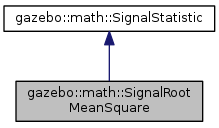##gazebo::math::SignalRootMeanSquare Class Reference

Computing the square root of the mean squared value of a discretely sampled signal. More...

`#include <math/gzmath.hh>`

Inheritance diagram for gazebo::math::SignalRootMeanSquare:[legend]

## Public Member Functions

virtual void InsertData (const double _data)
Add a new sample to the statistical measure. More...

virtual std::string ShortName () const
Get a short version of the name of this statistical measure. More...

virtual double Value () const
Get the current value of the statistical measure. More...Public Member Functions inherited from gazebo::math::SignalStatistic
SignalStatistic ()
Constructor. More...

virtual ~SignalStatistic ()
Destructor. More...

virtual size_t Count () const
Get number of data points in measurement. More...

virtual void Reset ()
Forget all previous data. More...Protected Attributes inherited from gazebo::math::SignalStatistic
SignalStatisticPrivatedataPtr
Pointer to private data. More...

## Detailed Description

Computing the square root of the mean squared value of a discretely sampled signal.

## Member Function Documentation

 virtual void gazebo::math::SignalRootMeanSquare::InsertData ( const double _data )
virtual

Add a new sample to the statistical measure.

Parameters
 [in] _data New signal data point.

Implements gazebo::math::SignalStatistic.

 virtual std::string gazebo::math::SignalRootMeanSquare::ShortName ( ) const
virtual

Get a short version of the name of this statistical measure.

Returns
"rms"

Implements gazebo::math::SignalStatistic.

 virtual double gazebo::math::SignalRootMeanSquare::Value ( ) const
virtual

Get the current value of the statistical measure.

Returns
Current value of the statistical measure.

Implements gazebo::math::SignalStatistic.

The documentation for this class was generated from the following file: